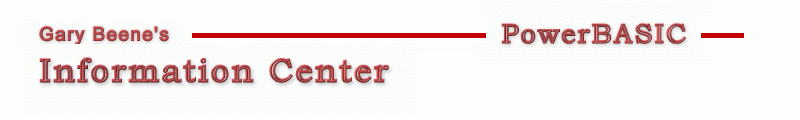## Min/Max Value of Array

Category: Arrays

Date: 03-28-2012

'PowerBASIC has no built-in function which tells you the min
'and max values contained in an array.  Here's a simple approach.

'Primary Code:
'This code simply loops through each element of the array. The min/max
'values must first be set to any value contained in the array.

LoValue = MyArray(LBound(MyArray)) : HiValue = LoValue
For i = LBound(MyArray) To UBound(MyArray)
If LoValue < MyArray(i) Then LoValue = MyArray(i)
If HiValue > MyArray(i) Then HiValue = MyArray(i)
Next i

'Compilable Example:
#Compiler PBWin 9, PBWin 10
#Compile EXE
#Dim All
#Include "Win32API.inc"
Global hDlg as DWord

Function PBMain() As Long
Dialog New Pixels, 0, "Test Code",300,300,200,200, %WS_OverlappedWindow To hDlg
Control Add Button, hDlg, 100,"Push", 50,10,100,20
Dialog Show Modal hDlg Call DlgProc
End Function

CallBack Function DlgProc() As Long
If CB.Msg = %WM_Command AND CB.Ctl = 100 AND CB.Ctlmsg = %BN_Clicked Then
Local LoValue, HiValue, i As Long
'create/populate test array
Dim MyArray(5 to 100) As Long
Randomize : For i = LBound(MyArray) To UBound(MyArray) : MyArray(i) = Rnd(0,1000000) : Next i

'Get min/max values
LoValue = MyArray(LBound(MyArray)) : HiValue = LoValue
For i = LBound(MyArray) To UBound(MyArray)
If LoValue < MyArray(i) Then LoValue = MyArray(i)
If HiValue > MyArray(i) Then HiValue = MyArray(i)
Next i
MsgBox "Min: " + Str\$(LoValue) + "    Max: " + Str\$(HiValue)
End If
End Function

'gbs_00427
'Date: 03-10-2012

created by gbSnippets
http://www.garybeene.com/sw/gbsnippets.htm